The Language of the Builders

Much has was written about the Great Pyramid's dimensions with the "official"  royal cubit of 52.36 cm, but it's not with that one I'll show you and reveals incredible things, but with cubits many are unaware and which it was kept secret...Because the Egyptians had other three cubits... (source)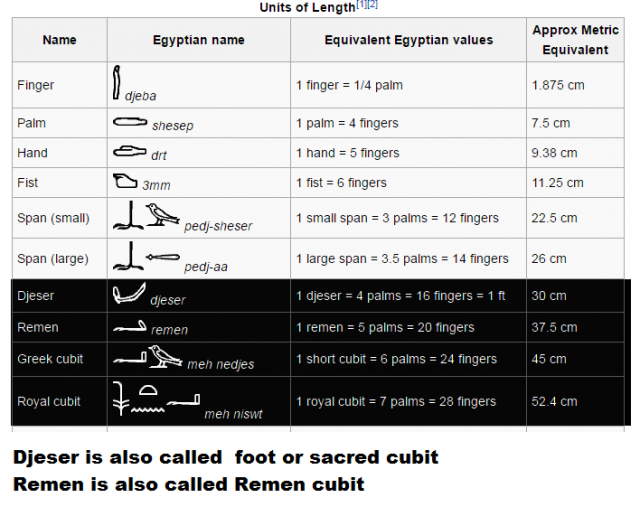The official Egyptologists speak only of the royal cubit, which she could not remain secret, just as it is in the dimensions of the King's Chamber and therefore, we can not erase it.

As I have shown in another  article The Return Of Planet X Register On \$ 1 Bill these "new" cubits open incredible doors, for example, they prove a mathematical way that the Ark of the covenant was in the trunk of granite who is in the King's chamber  of the pyramid  and therefore that  "God" and the pyramid were linked.

I'll show you in this article, that these new cubits revealed about the dimensions of the Great Pyramid and you'll see, it's pretty amazing...

To begin, take the two most famous numbers of the universe...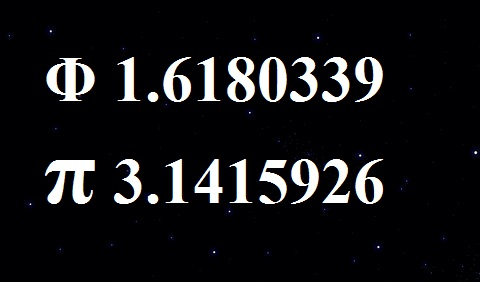Phi, the number of God 1.618... and Pi 3.1415...

divide these two numbers...

You get 0.515036

Now, going on wikipedia and see the dimensions of the pyramid ;
https://en.wikipedia.org/wiki/Great_Pyramid_of_Giza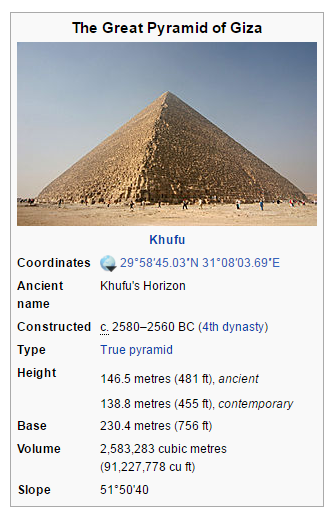look at the value of the slope...Incredible, right ?

But it is not finished.

For the meaning of dimensions we calculate with two digits after the decimal point for the cubits, because we are talking about symbolism.

The length of this edge, which rises to the top of the pyramid with this slope is 186.52m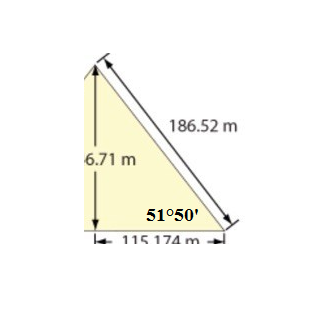divide that length with value of 0.51

You get 365.72  which is approximately the number of days in a solar year!

If you lower the outdoor temperature of 10°, you reduce the height of the pyramid and you get a very close value of 365.25

0.51 corresponds to the diameter of a circle which has a circumference 1.618, and we can call this cubit,  cubit of circle or cubit of the sun.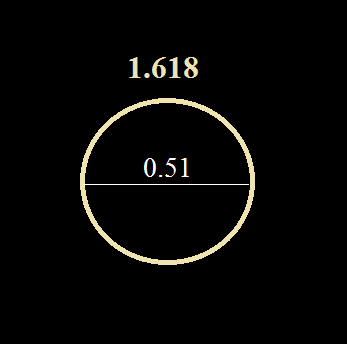We will continue the argument with the square.

The side of a square perimeter for 1.618 is 0.40
I'll call this cubit of the square or cubit of the moon.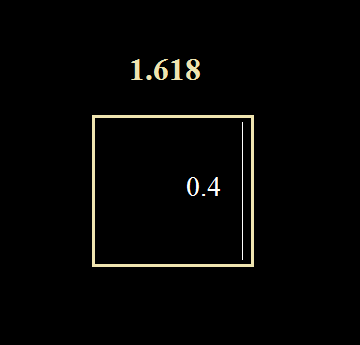We continue the argument with the triangle but this time in a more symbolic way ...
Life on Earth is possible thanks to the sun and the moon.

I'm going to marry the sun and the moon to get the third cubit, that of the triangle or the cubit of the Earth .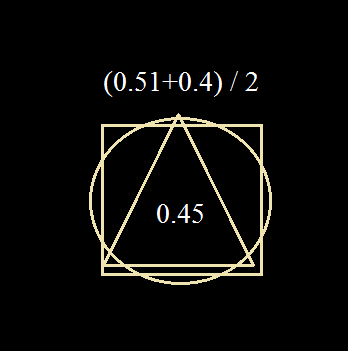It reminds us of a very famous thing...This value corresponds with the Greek cubit that you can see in the photo above.

Now look at the dimensions of the base of the pyramid :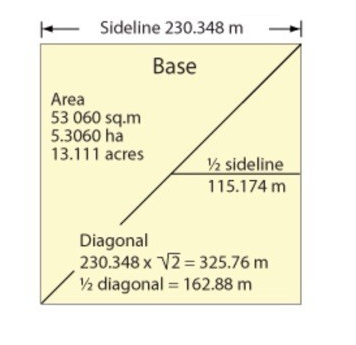If I converted 230.34m in cubit of the circle (0.51), we get about 451 Cubits.

The first thought, these 451 cubits did nothing special, but you'll see that it's incredible...

In a previous article "Decoding steps of the pyramid"  I explained a clear logical and coherent interpretation of the measurements of the Pyramid of Cheops.
Unfortunately there was a problem at the base dimension for it to work in a perfect manner.
For the base I got a value for one side of 451 then we had to have 450 to be exact in reasoning.
As this could not be a mistake of the builders so I thought it probably came from a poor estimate of the base meter...

But there is another possibility...

The builders did not want 450 but 451 !
451 is not a mistake but a will.

I'll let you watch the video which is an incredible proof that some knew and used the code  of the builders for their secret society.Go to 9'

It is a LANGUAGE !

They speaks with mathematics and geometry, the universal language.

Now try to understand the meaning of 451 with this language.

You will see how our builders are highly intelligent ...

We should have had 450, so we can write 451 this way:

450 + 1

There are two messages:

The first is the simplest, the "one" is too, so it means an anomaly.
I will come back later...

The second :

450 + 1

As it is a language so I'll add.

The sum of the digits = 9 and we get now:

450  1  9

Now multiply by 4 to our perimeter:
(Recall 450  1  9  is the value of one side of the "square" that is the base of our pyramid)

1800   4  36

take the Zero of  1800 and give it to 36

180 4  360

180 = 180° of the triangle (the sum of the three angles of a triangle always 180°).

4     = the four sides of the square.

360 = 360° of a circle.With +1, the dimensions of the pyramid symbolize, as three cubits the three basic geometrical figures: triangle, square and circle.

Continue in decoding:If I convert the 450 cubits of my base with the cubit of the triangle or of the Earth (0.45) I get exactly 1,000.

So we have a perfect square of side 1000 and a perimeter of 4000!

And as we have seen that the base also symbolizes a circle, so we also have a circumference of circle 4000.

I took three days to understand the meaning of the 4000 ...

It's just the Earth to scale 1/10th!

Circumference of the earth by the Poles (the equator undergoes centrifugal force) is 40.000km (about 7km).

The pyramid represents our planet with its dimensions.

Excerpt from the book For Them v1.618:

The southern circumference to the poles of our planet is 40 000 km (about 7 km).
Strange…
The circumference of the circle (the earth) = perimeter perfect square.
This beautiful coincidence can only lead us to represent us the Earth by the square.
This is what the builders have done or rather, wanted ...
They put their name to an measure and have included this measure in the pyramid.
Then the men took the measure in the pyramid and now they gives us the name!
An invisible and indelible ink, what intelligence, what genius! "The Earth is a square (symbol of the house) by its dimensions, a sphere by its nature. The Pyramid is a perfect union of these two figures.

Everything is clear.

The Pyramid symbolizes our planet, the Earth, our Home...450 + 1

Decomposed in another way:

45 0 1

45 = cubit triangle, the Earth

It remains the 0 and the 1, see the article 'The amazing discovery' for the explanation
The anomaly is the Planet X that I discovered only in the month of April 2015.

And I gave you and explained the date of return of this planet, in this article and in the video above.